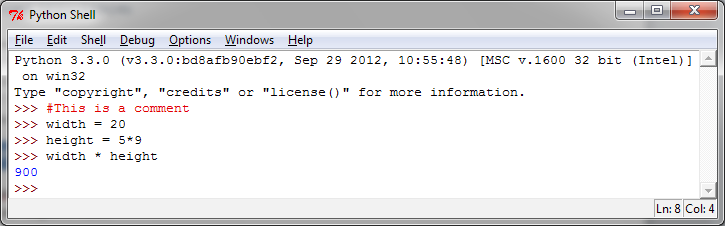# 7. 介绍Python¶

Python是一种动态的解释型语言，应用场景广泛。它具有以下特性：

• 简明、易读的语法
• 丰富的标准库。通过第三方的软件模块，我们可以方便地添加数据类型、函数和对象
• 上手简单，开发和调试速度快。Python代码的开发速度可能比C/C++快10倍
• 基于Exception的错误处理机制
• 强大的自省功能
• 丰富的文档和活跃的社区

Python也可以作为一种胶水语言。通过Python，擅长不同编程语言的程序员可以在同一个项目中合作。例如开发一个数据型的应用时，C/C++程序员可以从底层实现高效的数值计算算法，而数据科学家可以通过Python调用这些算法，而不用花时间去学习底层的编程语言，C/C++程序员也不需要去理解科学数据层面的东西。

## 7.2. 如何做…¶

• 整数类型的操作：

```>>> # This is a comment
>>> width = 20
>>> height = 5*9
>>> width * height
900
```

鉴于这是我们第一次展示代码，下面贴一下代码在Python解释器中的样子:• 复数(译者注：这里原书 `abs(a) = 5` ，应该是错了）：

```>>> a=1.5+0.5j
>>> a.real
1.5
>>> a.imag
0.5
>>> abs(a)  # sqrt(a.real**2 + a.imag**2)
1.5811388300841898
```
• 字符串操作：

```>>> word = 'Help' + 'A' >>> word
'HelpA'
>>> word
'A'
>>> word[0:2]
'He'
>>> word[-1]  # 最后一个字符
'A'
```
• 列表（list）操作：

```>>> a = ['spam', 'eggs', 100, 1234] >>> a
'spam'
>>> a
1234
>>> a[-2]
100
>>> a[1:-1]
['eggs', 100]
>>> len(a)
4
```
• `while` 循环：

```# Fibonacci series:
>>> while b < 10:
...     print b
...     a, b = b, a+b
...
1
1
2
3
5
8
```
• `if` 命令： 首先我们用 `input()` 从键盘读入一个整数：

```>>>x = int(input("Please enter an integer here: "))
```

然后在输入的数字中使用 `if` 进行判断：

```>>>if x < 0:
...      print ('the number is negative')
...elif x == 0:
...      print ('the number is zero')
...elif x == 1:
...      print ('the number is one')
...else:
...      print ('More')
...
```
• `for` 循环：:

```>>> # Measure some strings:
... a = ['cat', 'window', 'defenestrate'] >>> for x in a:
... print (x, len(x))
...
cat 3
window 6
defenestrate 12
```
• 定义函数：

```>>> def fib(n):  # 生成n以内的菲波那切数列
...    """Print a Fibonacci series up to n."""
...    a, b = 0, 1
...    while b < n:
...        print(b),
...        a, b = b, a+b
>>> # Now call the function we just defined:
... fib(2000)
1 1 2 3 5 8 13 21 34 55 89 144 233 377 610 987 1597
```
• 导入模块：

```>>> import math
>>> math.sin(1)
0.8414709848078965
>>> from math import *
>>> log(1)
0.0
```
• 定义类：

```>>> class Complex:
...     def __init__(self, realpart, imagpart):
...         self.r = realpart
...         self.i = imagpart
...
>>> x = Complex(3.0, -4.5)
>>> x.r, x.i
(3.0, -4.5)
```